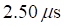Chapter 12, Problem 4P

Chapter
Section
Textbook Problem

A computer in a laboratory requiresto make a certain calculation, as measured by a scientist in the lab. To someone moving past the lab at a relative speed of 0.995c, how long will the same calculation take?

To determine

To someone moving past the lab at a relative speed of 0.995c, how long will the same calculation take?

Explanation

Given info:

A computer in a laboratory requires 2.50 p,s to make a certain calculation, as measured by a scientist in the lab.

Formula used:

Using the formula of time dilation.

Δt=Δt1 v 2 c 2

Here v is the speed of particle and c is speed of light.

Calculation:

We have, Δt=2.5×106sand v= 0.995c

Substituting the given values in above formula, we get

Δt=2

Still sussing out bartleby?

Check out a sample textbook solution.

See a sample solution

The Solution to Your Study Problems

Bartleby provides explanations to thousands of textbook problems written by our experts, many with advanced degrees!

Get Started